# Inroduction to Gaussian Processes¶

## Machine Learning Summer School, Sydney, Australia¶

### Neil D. Lawrence¶

When we form a Gaussian process we assume data is jointly Gaussian with a particular mean and covariance, $$\mathbf{y}|\mathbf{X} \sim \mathcal{N}(\mathbf{m}(\mathbf{X}), \mathbf{K}(\mathbf{X})),$$ where the conditioning is on the inputs $\mathbf{X}$ which are used for computing the mean and covariance. For this reason they are known as mean and covariance functions.

In :
%matplotlib inline
import numpy as np
import scipy as sp
import matplotlib.pyplot as plt
import pods

/Users/neil/Library/Enthought/Canopy_64bit/User/lib/python2.7/site-packages/pytz/__init__.py:29: UserWarning: Module pods was already imported from /Users/neil/sods/ods/pods/__init__.py, but /Users/neil/Library/Enthought/Canopy_64bit/User/lib/python2.7/site-packages is being added to sys.path
from pkg_resources import resource_stream


## Marginal Likelihood¶

To understand the Gaussian process we're going to build on our understanding of the marginal likelihood for Bayesian regression. In the session on Bayesian regression we sampled directly from the weight vector, $\mathbf{w}$ and applied it to the basis matrix $\boldsymbol{\Phi}$ to obtain a sample from the prior and a sample from the posterior. It is often helpful to think of modeling techniques as generative models. To give some thought as to what the process for obtaining data from the model is. From the perspective of Gaussian processes, we want to start by thinking of basis function models, where the parameters are sampled from a prior, but move to thinking about sampling from the marginal likelihood directly.

## Sampling from the Prior¶

The first thing we'll do is to set up the parameters of the model, these include the parameters of the prior, the parameters of the basis functions and the noise level.

In :
# set prior variance on w
alpha = 4.
# set the order of the polynomial basis set
degree = 5
# set the noise variance
sigma2 = 0.01


Now we have the variance, we can sample from the prior distribution to see what form we are imposing on the functions a priori.

Let's now compute a range of values to make predictions at, spanning the new space of inputs,

In :
def polynomial(x, degree, loc, scale):
degrees = np.arange(degree+1)
return ((x-loc)/scale)**degrees


now let's build the basis matrices. First we load in the data

In :
data = pods.datasets.olympic_marathon_men()
x = data['X']
y = data['Y']

In :
plt.plot(x, y, 'rx')

Out:
[<matplotlib.lines.Line2D at 0x10de2f610>]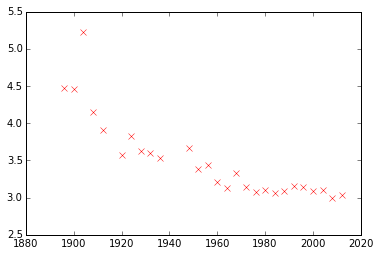In :
scale = np.max(x) - np.min(x)
loc = np.min(x) + 0.5*scale

num_data = x.shape
num_pred_data = 100 # how many points to use for plotting predictions
x_pred = np.linspace(1880, 2030, num_pred_data)[:, None] # input locations for predictions
Phi_pred = polynomial(x_pred, degree=degree, loc=loc, scale=scale)
Phi = polynomial(x, degree=degree, loc=loc, scale=scale)


### Weight Space View¶

To generate typical functional predictions from the model, we need a set of model parameters. We assume that the parameters are drawn independently from a Gaussian density, $$\mathbf{w} \sim \mathcal{N}(\mathbf{0}, \alpha\mathbf{I}),$$ then we can combine this with the definition of our prediction function $f(\mathbf{x})$, $$f(\mathbf{x}) = \mathbf{w}^\top \boldsymbol{\phi}(\mathbf{x}).$$ We can now sample from the prior density to obtain a vector $\mathbf{w}$ using the function np.random.normal and combine these parameters with our basis to create some samples of what $f(\mathbf{x})$ looks like,

In :
num_samples = 10
K = degree+1
for i in xrange(num_samples):
z_vec = np.random.normal(size=(K, 1))
w_sample = z_vec*np.sqrt(alpha)
f_sample = np.dot(Phi_pred,w_sample)
plt.plot(x_pred, f_sample)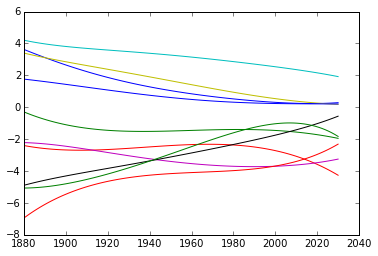### Function Space View¶

The process we have used to generate the samples is a two stage process. To obtain each function, we first generated a sample from the prior, $$\mathbf{w} \sim \mathcal{N}(\mathbf{0}, \alpha \mathbf{I})$$ then if we compose our basis matrix, $\boldsymbol{\Phi}$ from the basis functions associated with each row then we get, $$\mathbf{\Phi} = \begin{bmatrix}\boldsymbol{\phi}(\mathbf{x}_1) \\ \vdots \\ \boldsymbol{\phi}(\mathbf{x}_n)\end{bmatrix}$$ then we can write down the vector of function values, as evaluated at $$\mathbf{f} = \begin{bmatrix} f_1 \\ \vdots \\ f_n\end{bmatrix}$$ in the form $$\mathbf{f} = \boldsymbol{\Phi} \mathbf{w}.$$

Now we can use standard properties of multivariate Gaussians to write down the probability density that is implied over $\mathbf{f}$. In particular we know that if $\mathbf{w}$ is sampled from a multivariate normal (or multivariate Gaussian) with covariance $\alpha \mathbf{I}$ and zero mean, then assuming that $\boldsymbol{\Phi}$ is a deterministic matrix (i.e. it is not sampled from a probability density) then the vector $\mathbf{f}$ will also be distributed according to a zero mean multivariate normal as follows, $$\mathbf{f} \sim \mathcal{N}(\mathbf{0},\alpha \boldsymbol{\Phi} \boldsymbol{\Phi}^\top).$$

The question now is, what happens if we sample $\mathbf{f}$ directly from this density, rather than first sampling $\mathbf{w}$ and then multiplying by $\boldsymbol{\Phi}$. Let's try this. First of all we define the covariance as $$\mathbf{K} = \alpha \boldsymbol{\Phi}\boldsymbol{\Phi}^\top.$$

In :
K = alpha*np.dot(Phi_pred, Phi_pred.T)


Now we can use the np.random.multivariate_normal command for sampling from a multivariate normal with covariance given by $\mathbf{K}$ and zero mean,

In :
for i in np.arange(10):
f_sample = np.random.multivariate_normal(mean=np.zeros(x_pred.size),
cov=K)
plt.plot(x_pred.flatten(), f_sample.flatten())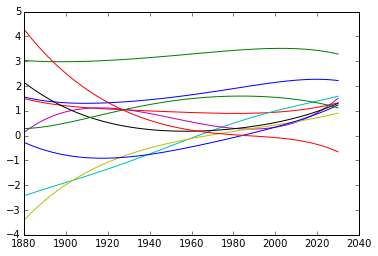The samples appear very similar to those which we obtained indirectly. That is no surprise because they are effectively drawn from the same mutivariate normal density. However, when sampling $\mathbf{f}$ directly we created the covariance for $\mathbf{f}$. We can visualise the form of this covaraince in an image in python with a colorbar to show scale.

In :
fig, ax = plt.subplots(figsize=(8,8))
im = ax.imshow(K, interpolation='none')
fig.colorbar(im)

Out:
<matplotlib.colorbar.Colorbar instance at 0x10f21ee18>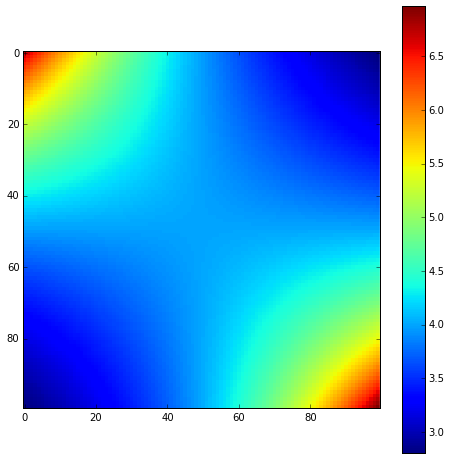This image is the covariance expressed between different points on the function. In regression we normally also add independent Gaussian noise to obtain our observations $\mathbf{y}$, $$\mathbf{y} = \mathbf{f} + \boldsymbol{\epsilon}$$ where the noise is sampled from an independent Gaussian distribution with variance $\sigma^2$, $$\epsilon \sim \mathcal{N}(\mathbf{0}, \sigma^2 \mathbf{I}).$$ we can use properties of Gaussian variables, i.e. the fact that sum of two Gaussian variables is also Gaussian, and that it's covariance is given by the sum of the two covariances, whilst the mean is given by the sum of the means, to write down the marginal likelihood, $$\mathbf{y} \sim \mathcal{N}(\mathbf{0}, \alpha \boldsymbol{\Phi}\boldsymbol{\Phi}^\top + \sigma^2\mathbf{I}).$$ Sampling directly from this density gives us the noise corrupted functions,

In :
K = alpha*np.dot(Phi_pred, Phi_pred.T) + sigma2*np.eye(x_pred.size)
for i in xrange(10):
y_sample = np.random.multivariate_normal(mean=np.zeros(x_pred.size), cov=K)
plt.plot(x_pred.flatten(), y_sample.flatten())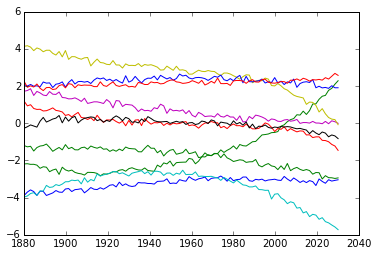where the effect of our noise term is to roughen the sampled functions, we can also increase the variance of the noise to see a different effect,

In :
sigma2 = 1.
K = alpha*np.dot(Phi_pred, Phi_pred.T) + sigma2*np.eye(x_pred.size)
for i in xrange(10):
y_sample = np.random.multivariate_normal(mean=np.zeros(x_pred.size), cov=K)
plt.plot(x_pred.flatten(), y_sample.flatten())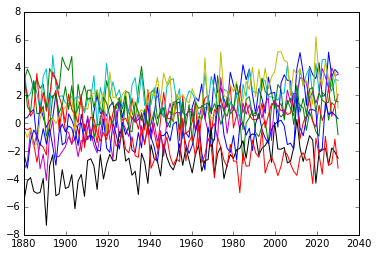### Function Space Reflection¶

How do you include the noise term when sampling in the weight space point of view?

## Gaussian Process¶

In our session on Bayesian regression we sampled from the prior over parameters. Through the properties of multivariate Gaussian densities this prior over parameters implies a particular density for our data observations, $\mathbf{y}$. In this session we sampled directly from this distribution for our data, avoiding the intermediate weight-space representation. This is the approach taken by Gaussian processes. In a Gaussian process you specify the covariance function directly, rather than implicitly through a basis matrix and a prior over parameters. Gaussian processes have the advantage that they can be nonparametric, which in simple terms means that they can have infinite basis functions. In the lectures we introduced the exponentiated quadratic covariance, also known as the RBF or the Gaussian or the squared exponential covariance function. This covariance function is specified by $$k(\mathbf{x}, \mathbf{x}^\prime) = \alpha \exp\left( -\frac{\left\Vert \mathbf{x}-\mathbf{x}^\prime\right\Vert^2}{2\ell^2}\right).$$ where $\left\Vert\mathbf{x} - \mathbf{x}^\prime\right\Vert^2$ is the squared distance between the two input vectors $$\left\Vert\mathbf{x} - \mathbf{x}^\prime\right\Vert^2 = (\mathbf{x} - \mathbf{x}^\prime)^\top (\mathbf{x} - \mathbf{x}^\prime)$$ Let's build a covariance matrix based on this function. First we define the form of the covariance function,

In :
def exponentiated_quadratic(x, x_prime, variance, lengthscale):
squared_distance = ((x-x_prime)**2).sum()
return variance*np.exp((-0.5*squared_distance)/lengthscale**2)


We can use this to compute directly the covariance for $\mathbf{f}$ at the points given by x_pred. Let's define a new function K() which does this,

In :
def compute_kernel(X, X2, kernel, **kwargs):
K = np.zeros((X.shape, X2.shape))
for i in np.arange(X.shape):
for j in np.arange(X2.shape):
K[i, j] = kernel(X[i, :], X2[j, :], **kwargs)

return K


Now we can image the resulting covariance,

In :
K = compute_kernel(x_pred, x_pred, exponentiated_quadratic, variance=1., lengthscale=10.)


To visualise the covariance between the points we can use the imshow function in matplotlib.

In :
fig, ax = plt.subplots(figsize=(8,8))
im = ax.imshow(K, interpolation='none')
fig.colorbar(im)

Out:
<matplotlib.colorbar.Colorbar instance at 0x10f26ba28>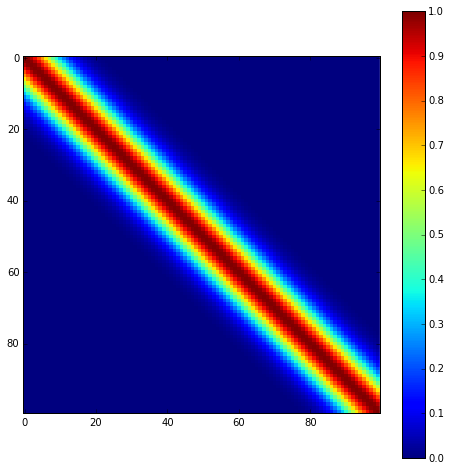Finally, we can sample functions from the marginal likelihood.

In :
for i in xrange(10):
y_sample = np.random.multivariate_normal(mean=np.zeros(x_pred.size), cov=K)
plt.plot(x_pred.flatten(), y_sample.flatten())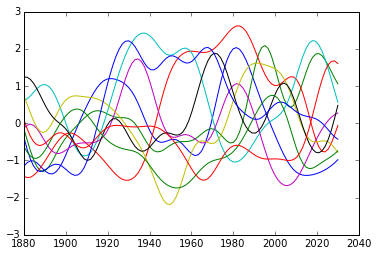### Exercise: Moving Parameters¶

Have a play with the parameters for this covariance function (the lengthscale and the variance) and see what effects the parameters have on the types of functions you observe.

In :



## Gaussian Process¶

The Gaussian process perspective takes the marginal likelihood of the data to be a joint Gaussian density with a covariance given by $\mathbf{K}$. So the model likelihood is of the form, $$p(\mathbf{y}|\mathbf{X}) = \frac{1}{(2\pi)^{\frac{n}{2}}|\mathbf{K}|^{\frac{1}{2}}} \exp\left(-\frac{1}{2}\mathbf{y}^\top \left(\mathbf{K}+\sigma^2 \mathbf{I}\right)^{-1}\mathbf{y}\right)$$ where the input data, $\mathbf{X}$, influences the density through the covariance matrix, $\mathbf{K}$ whose elements are computed through the covariance function, $k(\mathbf{x}, \mathbf{x}^\prime)$.

This means that the negative log likelihood (the objective function) is given by, $$E(\boldsymbol{\theta}) = \frac{1}{2} \log |\mathbf{K}| + \frac{1}{2} \mathbf{y}^\top \left(\mathbf{K} + \sigma^2\mathbf{I}\right)^{-1}\mathbf{y}$$ where the parameters of the model are also embedded in the covariance function, they include the parameters of the kernel (such as lengthscale and variance), and the noise variance, $\sigma^2$.

Let's create a class in python for storing these variables.

In :
class GP():
def __init__(self, X, y, sigma2, kernel, **kwargs):
self.K = compute_kernel(X, X, kernel, **kwargs)
self.X = X
self.y = y
self.sigma2 = sigma2
self.kernel = kernel
self.kernel_args = kwargs
self.update_inverse()

def update_inverse(self):
# Preompute the inverse covariance and some quantities of interest
## NOTE: This is not the correct *numerical* way to compute this! It is for ease of use.
self.Kinv = np.linalg.inv(self.K+self.sigma2*np.eye(self.K.shape))
# the log determinant of the covariance matrix.
self.logdetK = np.linalg.det(self.K+self.sigma2*np.eye(self.K.shape))
# The matrix inner product of the inverse covariance
self.Kinvy = np.dot(self.Kinv, self.y)
self.yKinvy = (self.y*self.Kinvy).sum()

def log_likelihood(self):
# use the pre-computes to return the likelihood
return -0.5*(self.K.shape*np.log(2*np.pi) + self.logdetK + self.yKinvy)

def objective(self):
# use the pre-computes to return the objective function
return -self.log_likelihood()


## Making Predictions¶

We now have a probability density that represents functions. How do we make predictions with this density? The density is known as a process because it is consistent. By consistency, here, we mean that the model makes predictions for $\mathbf{f}$ that are unaffected by future values of $\mathbf{f}^*$ that are currently unobserved (such as test points). If we think of $\mathbf{f}^*$ as test points, we can still write down a joint probability density over the training observations, $\mathbf{f}$ and the test observations, $\mathbf{f}^*$. This joint probability density will be Gaussian, with a covariance matrix given by our covariance function, $k(\mathbf{x}_i, \mathbf{x}_j)$. $$\begin{bmatrix}\mathbf{f} \\ \mathbf{f}^*\end{bmatrix} \sim \mathcal{N}\left(\mathbf{0}, \begin{bmatrix} \mathbf{K} & \mathbf{K}_\ast \\ \mathbf{K}_\ast^\top & \mathbf{K}_{\ast,\ast}\end{bmatrix}\right)$$ where here $\mathbf{K}$ is the covariance computed between all the training points, $\mathbf{K}_\ast$ is the covariance matrix computed between the training points and the test points and $\mathbf{K}_{\ast,\ast}$ is the covariance matrix computed betwen all the tests points and themselves. To be clear, let's compute these now for our example, using x and y for the training data (although y doesn't enter the covariance) and x_pred as the test locations.

In :
# set covariance function parameters
variance = 16.0
lengthscale = 32
# set noise variance
sigma2 = 0.05

K = compute_kernel(x, x, exponentiated_quadratic, variance=variance, lengthscale=lengthscale)
K_star = compute_kernel(x, x_pred, exponentiated_quadratic, variance=variance, lengthscale=lengthscale)
K_starstar = compute_kernel(x_pred, x_pred, exponentiated_quadratic, variance=variance, lengthscale=lengthscale)


Now we use this structure to visualise the covariance between test data and training data. This structure is how information is passed between trest and training data. Unlike the maximum likelihood formalisms we've been considering so far, the structure expresses correlation between our different data points. However, we now have a joint density between some variables of interest. In particular we have the joint density over $p(\mathbf{f}, \mathbf{f}^*)$. The joint density is Gaussian and zero mean. It is specified entirely by the covariance matrix, $\mathbf{K}$. That covariance matrix is, in turn, defined by a covariance function. Now we will visualise the form of that covariance in the form of the matrix, $$\begin{bmatrix} \mathbf{K} & \mathbf{K}_\ast \\ \mathbf{K}_\ast^\top & \mathbf{K}_{\ast,\ast}\end{bmatrix}$$

In :
fig, ax = plt.subplots(figsize=(8,8))
im = ax.imshow(np.vstack([np.hstack([K, K_star]), np.hstack([K_star.T, K_starstar])]), interpolation='none')
# Add lines for separating training and test data
ax.axvline(x.shape-1, color='w')
ax.axhline(x.shape-1, color='w')
fig.colorbar(im)

Out:
<matplotlib.colorbar.Colorbar instance at 0x10e197b48>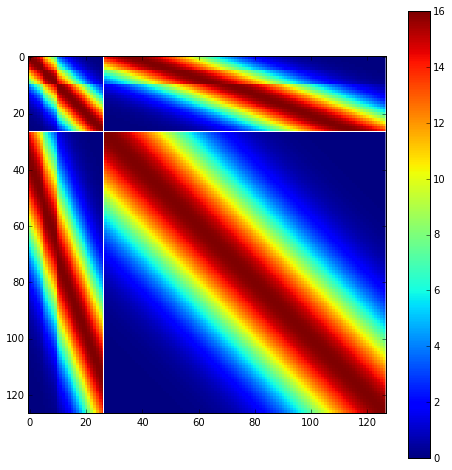There are four blocks to this color plot. The upper left block is the covariance of the training data with itself, $\mathbf{K}$. We see some structure here due to the missing data from the first and second world wars. Alongside this covariance (to the right and below) we see the cross covariance between the training and the test data ($\mathbf{K}_*$ and $\mathbf{K}_*^\top$). This is giving us the covariation between our training and our test data. Finally the lower right block The banded structure we now observe is because some of the training points are near to some of the test points. This is how we obtain 'communication' between our training data and our test data. If there is no structure in $\mathbf{K}_*$ then our belief about the test data simply matches our prior.

## Conditional Density¶

Just as in naive Bayes, we first defined the joint density (although there it was over both the labels and the inputs, $p(\mathbf{y}, \mathbf{X})$ and now we need to define conditional distributions that answer particular questions of interest. In particular we might be interested in finding out the values of the function for the prediction function at the test data given those at the training data, $p(\mathbf{f}_*|\mathbf{f})$. Or if we include noise in the training observations then we are interested in the conditional density for the prediction function at the test locations given the training observations, $p(\mathbf{f}^*|\mathbf{y})$.

As ever all the various questions we could ask about this density can be answered using the sum rule and the product rule. For the multivariate normal density the mathematics involved is that of linear algebra, with a particular emphasis on the partitioned inverse or block matrix inverse, but they are beyond the scope of this course, so you don't need to worry about remembering them or rederiving them. We are simply writing them here because it is this conditional density that is necessary for making predictions.

The conditional density is also a multivariate normal, $$\mathbf{f}^* | \mathbf{y} \sim \mathcal{N}(\boldsymbol{\mu}_f,\mathbf{C}_f)$$ with a mean given by $$\boldsymbol{\mu}_f = \mathbf{K}_*^\top \left[\mathbf{K} + \sigma^2 \mathbf{I}\right]^{-1} \mathbf{y}$$ and a covariance given by $$\mathbf{C}_f = \mathbf{K}_{*,*} - \mathbf{K}_*^\top \left[\mathbf{K} + \sigma^2 \mathbf{I}\right]^{-1} \mathbf{K}_\ast.$$ Let's compute what those posterior predictions are for the olympic marathon data.

In :
def posterior_f(self, X_test):
K_starstar = compute_kernel(X_test, X_test, self.kernel, **self.kernel_args)
K_star = compute_kernel(self.X, X_test, self.kernel, **self.kernel_args)
A = np.dot(self.Kinv, K_star)
mu_f = np.dot(A.T, y)
C_f = K_starstar - np.dot(A.T, K_star)
return mu_f, C_f

# attach the new method to class GP():
GP.posterior_f = posterior_f

In :
model = GP(x, y, sigma2, exponentiated_quadratic,
variance=variance, lengthscale=lengthscale)
mu_f, C_f = model.posterior_f(x_pred)


where for convenience we've defined

$$\mathbf{A} = \left[\mathbf{K} + \sigma^2\mathbf{I}\right]^{-1}\mathbf{K}_*.$$

We can visualize the covariance of the conditional,

In :
fig, ax = plt.subplots(figsize=(8,8))
im = ax.imshow(C_f, interpolation='none')
fig.colorbar(im)

Out:
<matplotlib.colorbar.Colorbar instance at 0x10f04c9e0>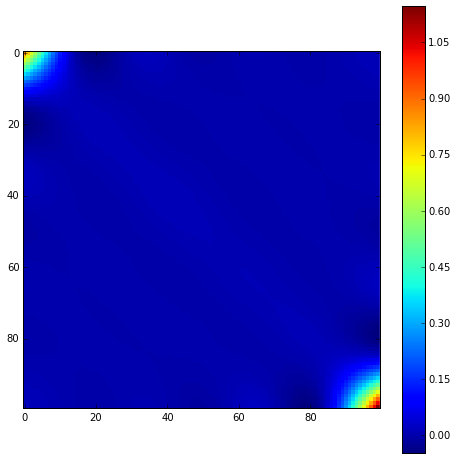and we can plot the mean of the conditional

In :
plt.plot(x, y, 'rx')
plt.plot(x_pred, mu_f, 'b-')

Out:
[<matplotlib.lines.Line2D at 0x10e4cfb50>]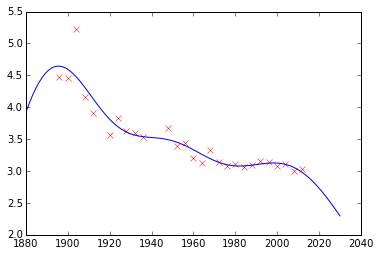as well as the associated error bars. These are given (similarly to the Bayesian parametric model from the last lab) by the standard deviations of the marginal posterior densities. The marginal posterior variances are given by the diagonal elements of the posterior covariance,

In :
var_f = np.diag(C_f)[:, None]
std_f = np.sqrt(var_f)


They can be added to the underlying mean function to give the error bars,

In :
plt.plot(x, y, 'rx')
plt.plot(x_pred, mu_f, 'b-')
plt.plot(x_pred, mu_f+2*std_f, 'b--')
plt.plot(x_pred, mu_f-2*std_f, 'b--')

Out:
[<matplotlib.lines.Line2D at 0x10f258050>]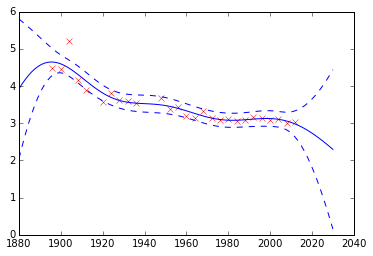This gives us a prediction from the Gaussian process. Remember machine learning is $$\text{data} + \text{model} \rightarrow \text{prediction}.$$ Here our data is from the olympics, and our model is a Gaussian process with two parameters. The assumptions about the world are encoded entirely into our Gaussian process covariance. The GP covariance assumes that the function is highly smooth, and that correlation falls off with distance (scaled according to the length scale, $\ell$). The model sustains the uncertainty about the function, this means we see an increase in the size of the error bars during periods like the 1st and 2nd World Wars when no olympic marathon was held.

## Exercises¶

Now try changing the parameters of the covariance function (and the noise) to see how the predictions change.

Now try sampling from this conditional density to see what your predictions look like. What happens if you sample from the conditional density in regions a long way into the future or the past? How does this compare with the results from the polynomial model?

## The Importance of the Covariance Function¶

The covariance function encapsulates our assumptions about the data. The equations for the distribution of the prediction function, given the training observations, are highly sensitive to the covariation between the test locations and the training locations as expressed by the matrix $\mathbf{K}_*$. We defined a matrix $\mathbf{A}$ which allowed us to express our conditional mean in the form, $$\boldsymbol{\mu}_f = \mathbf{A}^\top \mathbf{y},$$ where $\mathbf{y}$ were our training observations. In other words our mean predictions are always a linear weighted combination of our training data. The weights are given by computing the covariation between the training and the test data ($\mathbf{K}_*$) and scaling it by the inverse covariance of the training data observations, $\left[\mathbf{K} + \sigma^2 \mathbf{I}\right]^{-1}$. This inverse is the main computational object that needs to be resolved for a Gaussian process. It has a computational burden which is $O(n^3)$ and a storage burden which is $O(n^2)$. This makes working with Gaussian processes computationally intensive for the situation where $n>10,000$.

In :
from IPython.display import YouTubeVideo

Out:

## Improving the Numerics¶

In practice we shouldn't be using matrix inverse directly to solve the GP system. One more stable way is to compute the Cholesky decomposition of the kernel matrix. The log determinant of the covariance can also be derived from the Cholesky decomposition.

In :
def update_inverse(self):
# Perform Cholesky decomposition on matrix
self.R = sp.linalg.cholesky(self.K + self.sigma2*self.K.shape)
# compute the log determinant from Cholesky decomposition
self.logdetK = 2*np.log(np.diag(self.R)).sum()
# compute y^\top K^{-1}y from Cholesky factor
self.Rinvy = sp.linalg.solve_triangular(self.R, self.y)
self.yKinvy = (self.Rinvy**2).sum()

# compute the inverse of the upper triangular Cholesky factor
self.Rinv = sp.linalg.solve_triangular(self.R, np.eye(self.K.shape))
self.Kinv = np.dot(self.Rinv, self.Rinv.T)

GP.update_inverse = update_inverse

In :
for i in xrange(10):
y_sample = np.random.multivariate_normal(mean=mu_f.flatten(),
cov=C_f)
plt.plot(x_pred.flatten(), y_sample.flatten())
plt.plot(x, y, 'rx')

Out:
[<matplotlib.lines.Line2D at 0x10ea10f10>]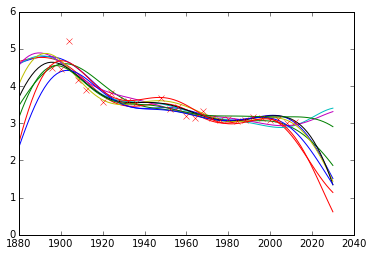## Capacity Control¶

Gaussian processes are sometimes seen as part of a wider family of methods known as kernel methods. Kernel methods are also based around covariance functions, but in the field they are known as Mercer kernels. Mercer kernels have interpretations as inner products in potentially infinite dimensional Hilbert spaces. This interpretation arises because, if we take $\alpha=1$, then the kernel can be expressed as $$\mathbf{K} = \boldsymbol{\Phi}\boldsymbol{\Phi}^\top$$ which imples the elements of the kernel are given by, $$k(\mathbf{x}, \mathbf{x}^\prime) = \boldsymbol{\phi}(\mathbf{x})^\top \boldsymbol{\phi}(\mathbf{x}^\prime).$$ So we see that the kernel function is developed from an inner product between the basis functions. Mercer's theorem tells us that any valid positive definite function can be expressed as this inner product but with the caveat that the inner product could be infinite length. This idea has been used quite widely to kernelize algorithms that depend on inner products. The kernel functions are equivalent to covariance functions and they are parameterized accordingly. In the kernel modeling community it is generally accepted that kernel parameter estimation is a difficult problem and the normal solution is to cross validate to obtain parameters. This can cause difficulties when a large number of kernel parameters need to be estimated. In Gaussian process modelling kernel parameter estimation (in the simplest case proceeds) by maximum likelihood. This involves taking gradients of the likelihood with respect to the parameters of the covariance function.

The easiest conceptual way to obtain the gradients is a two step process. The first step involves taking the gradient of the likelihood with respect to the covariance function, the second step involves considering the gradient of the covariance function with respect to its parameters. The relevant terms of the negative log likelihood are given by $$E(\boldsymbol{\theta}) = \frac{1}{2}\log |\hat{\mathbf{K}}| + \frac{1}{2} \mathbf{y}^\top \hat{\mathbf{K}}^{-1} \mathbf{y}$$ where $\hat{\mathbf{K}} = \mathbf{K} + \sigma^2 \mathbf{I}$ is the noise corrupted covariance matrix. The gradient with respect to that matrix can be computed as $$\frac{\text{d}E(\boldsymbol{\theta})}{\text{d}\hat{\mathbf{K}}} = \frac{1}{2}\hat{\mathbf{K}}^{-1} - \frac{1}{2}\hat{\mathbf{K}}^{-1}\mathbf{y}\mathbf{y}^\top \hat{\mathbf{K}}^{-1}$$ The can then be combined with gradients of the covariance function with respect to any parameters, $\frac{\text{d}\hat{\mathbf{K}}}{\text{d}\theta}$ using the chain rule. Mathematically that is written as $$\frac{\text{d}E(\boldsymbol{\theta})}{\text{d}\theta} = \text{tr}\left(\frac{\text{d}E(\boldsymbol{\theta})}{\text{d}\hat{\mathbf{K}}}\frac{\text{d}\hat{\mathbf{K}}}{\text{d}\theta}\right),$$ where the two gradient matrices are multiplied together. In in implementation, however, that is inefficient. It is more efficient to perform an element by element multiplication of the two matrices.

### Overall Process Scale¶

In general we won't be able to find parameters of the covariance function through fixed point equations, we will need to do graident based optimization, however there is one parameter that does have a fixed point update. Imagine the covariance $\hat{\mathbf{K}}$ has an overall scale such that $\hat{\mathbf{K}} = \alpha \boldsymbol{\Sigma}$. In this case the gradient of the covariance matrix with respect to $\alpha$ is simply $\mathbf{C}$ and $\hat{\mathbf{K}}^{-1}\mathbf{C} = \alpha^{-1}$. This means we can write, $$\frac{\text{d}E(\boldsymbol{\theta})}{\text{d}\alpha} = \frac{1}{2\alpha}\left(n - \frac{\mathbf{y}^\top\mathbf{C}^{-1}\mathbf{y}}{\alpha}\right)$$ which implies a fixed point updated is given by $$\alpha = \frac{\mathbf{y}^\top\mathbf{C}^{-1}\mathbf{y}}{n}.$$ The availability of a fixed point update for the overall scale means that sometimes, if we have a process of the form, $$\hat{\mathbf{K}} = \alpha \mathbf{K} + \sigma^2 \mathbf{I}$$ where $\mathbf{K}$ might be an exponentiated quadratic, or another covariance, that represents the signal, and $\sigma^2$ represents the contribution from the noise. Rather than representing directly in this form we might represent the model as $$\hat{\mathbf{K}} = \sigma^2\left(\hat{\alpha}\mathbf{K} + \mathbf{I})\right)$$ where $\hat{\alpha} = \frac{\alpha^2}{\sigma^2}$ is a signal to noise ratio. Although some care will need to be taken if the actual noise is very low as numerical instabilities are likely to occur.

## Capacity Control and Data Fit¶

The objective function can be decomposed into two terms, a capacity control term, and a data fit term. $$E(\boldsymbol{\theta}) = \frac{1}{2}\log |\hat{\mathbf{K}}| + \frac{1}{2} \mathbf{y}^\top \hat{\mathbf{K}}^{-1} \mathbf{y}$$ The capacity control term is the log determinant of the covariance, $\log |\hat{\mathbf{K}}|$. The data fit term is the matrix inner product between the data and the inverse covariance, $\frac{1}{2} \mathbf{y}^\top \hat{\mathbf{K}}^{-1} \mathbf{y}$.

The log determinant term has an interpretation as a log volume. The determinant of the covariance is related to Gaussian density's 'footprint'. Recall that the determinant is the product of the eigenvalues, and the eigenvalues represent a set of axes which describe the correlations of the Gaussian densities (the directions being given by the corresponding eigenvectors). The product of these eigenvalues gives the area (or volume) of the Gaussian density. Roughly speaking it represents the diversity of different functions the Gaussian process can represent. If the number is large, then the process can represent many functions. If it is small then the process can represent fewer functions. Although the terms 'many' and 'few' are badly defined here because the space is continuous and the Gaussian process represents a continuum of different functions. However, the intuition is maybe useful.

The data fit term is a little like a quadratic well, trying to locate the data at the lowest point. The data fit term can be driven to zero by increasing the scale of the covariance. However, this causes the capacity term to also increase, so a penalty is paid for this. Ideally, the data fit term should be reduced by matching correlations seen in the data with a corresponding correlation in the covariance function.

In :